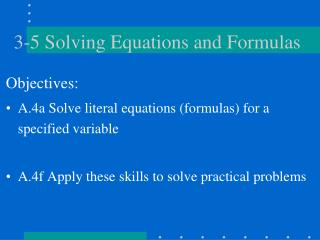DownloadDownload Presentation3-5 Solving Equations and Formulas

# 3-5 Solving Equations and Formulas

Download Presentation## 3-5 Solving Equations and Formulas

- - - - - - - - - - - - - - - - - - - - - - - - - - - E N D - - - - - - - - - - - - - - - - - - - - - - - - - - -
##### Presentation Transcript

1. 3-5 Solving Equations and Formulas Objectives: • A.4a Solve literal equations (formulas) for a specified variable • A.4f Apply these skills to solve practical problems

2. An equation is a mathematical sentence that contains an equal sign ( = ). Ex: x = yz What do x, y, and z stand for? A formula is an equation that states a rule for the relationship between certain quantities. Ex: A = lw What do A, l, and w stand for? Definitions:

3. What it means to solve: • To solve for x would mean to get x by itself on one side of the equation, with no x’s on the other side. (x = __ ) • Similarly, to solve for y would mean to get y by itself on one side of the equation, with no y’s on the other side. (y = __ )

4. 1) Solve the equation -5x + y = -56 for x. Ask yourself: What is the first thing being done to x, the variable being solved for? x is being multiplied by -5. DO UNDO ·-5 - y What is being done next? + y ÷(-5) y is being added to -5x. The DO-UNDO chart

5. First, subtract y from both sides of the equation. Next, divide by -5. This process actually requires LESS WORK than solving equations in one variable  Ex: -5x + y = -56 - y -y -5x = -56 - y -5 -5 x = -56 - y = 56 + y -5 5 Show all of your work!

6. Complete the do-undo chart. DO UNDO · 2 + 4y - 4y ÷ 2 To solve for x: First add 4y Then divide by 2 Ex: Solve 2x - 4y = 7 for x. 2x - 4y = 7 +4y + 4y 2x = 7 + 4y 2 2 x = 7 + 4y 2 This fraction cannot be simplified unless both terms in the numerator are divisible by 2. Let’s try another:

7. Solve a(y + 1) = b for y. DO UNDO + 1 ÷ a · a - 1 To solve for y: First divide by a Then subtract 1 a(y + 1) = b a a y + 1 = b a - 1 -1 y = b - 1 a Another example:

8. Solve 3ax - b = d - 4cx for x. First, we must get all terms with x together on one side. Add 4cx to both sides Add b to both sides Next, use the distributive property to factor x out of the two terms on the left. Now, x is being multiplied by (3a + 4c). To undo this, divide both sides by (3a + 4c). 3ax - b = d - 4cx +4cx +4cx 3ax - b + 4cx = d +b +b 3ax + 4cx = d + b x(3a + 4c) = d + b (3a + 4c) (3a + 4c) x = d + b (3a + 4c) Here’s a tricky one!

9. Try a few on your own. • Solve P = 1.2W for W. H2 • Solve P = 2l + 2w for l. • Solve 4x - 3m = 2mx - 5 for x.

10. DO UNDO · 1.2 · H2 ÷ H2 ÷ 1.2 W = PH2 1.2 DO UNDO · 2 -2w +2w ÷ 2 l = P - 2w 2 The answers: • Subtract 2mx, then • Add 3m to get • 4x - 2mx = 3m - 5 • x(4 - 2m) = 3m - 5 • Divide by (4 - 2m) • x = 3m - 5 • 4 - 2m